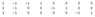# I Can't Solve This System

• WCMU101

#### WCMU101

Hey all. I'm not too sharp on linear algebra. I've done a first year university course on it, but that was a couple years ago & didn't go into much detail. Here is the problem:

Matrix I (n,1).
Matrix N (m,1).
Matrix G (n,m).

Now... I is a column vector of computed "double differences". These double differences are composed of original elements N. Matrix G relates the original elements to the double differences, such that:

G*N = I

G is composed of 1s and -1s, here is an example: (See attachment). So using that G:

n = 3, m = 8

So I already know the system can not be solved, since there are 8 unknowns (N) and only 3 equations. Hence I decided I needed more observations & I'll use least squares to get a solution. So for example:

[G G G]T*N = [I1 I2 I3]T

Where G is the same as it was above. And I1,2,3 correspond to the observations at time 1, 2 and 3 respectively. N is still 8x1.

So by least squares:

Let [G G G]T = G_Big

G_BigTG_Big*N = G_BigT[I1 I2 I3]T
N = (G_BigTG_Big)-1G_BigT[I1 I2 I3]T

Problem is (G_BigTG_Big)-1 is singular, I can't take the inverse. It happens for all sizes of G.

I don't know how else to solve this system.

Any help would be greatly appreciated!

Thanks. Nick.

#### Attachments

•matrix.jpg
1.7 KB · Views: 340